Study Materials:
CBSE NOTES ⇒ Class 10th ⇒ Science ⇒ 1. Chemical Reactions and Equations

# Balancing a Chemical Equation.

Here we would learn the method how to balance a chemical reaction.0

The method which we apply e.i hit and trail or inspecting method. Here we take Equation Fe + H2O → Fe3O4 + H2 as example I:

Steps;

(i)  Write each formula in box or imagine that each formula is in box. It is so that       you have to mind no changes should be made inside the box.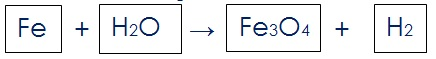(ii)  Count and list the numbers of atom of different element that present in                 unbalanced equation. Like this

Elements              Reactant                               Product

Fe                          1                                              3

O                           1                                              4

H                            2                                              2

First observe that what element has the maximum number of atoms. It may be from reactant and from product. Using this criteria we find that Fe3O4  is that compound and element is O that have maximum 4 atoms.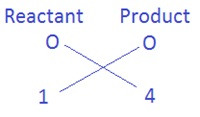To equalise the number of atoms of oxygen, we can put the coefficient 4 with H2O as 4H2O. Then we get the equation as,

Fe + 4H2O → Fe3O4 + H2

(iii)  The next maximum number of atoms having Fe. Which can be equalised

by same process.Putting the coefficient 3 with Fe as 3Fe in reactant side. Now, the equation    will be

3Fe + 4H2O → Fe3O4 + H2

(iv)  Finally we come to equalise Hydrogen atom of both side. We observe that          4H2 in reactant and H2 in product. Total atom of hydrogen in reactant side           is 4 × 2 = 8 and 2 in product. Then,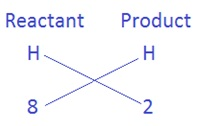Here we cannot put 2 on LHS and 8 on RHS. (8 ÷ 2 ) =4 , the coefficient will be 4 that equalises hydrogen atom on RHS. So finally equation will be.

3Fe + 4H2O → Fe3O4 + 4H2        (balanced equation)

Example II. We take,

HNO3 + Ca(OH)2 → Ca(NO3)2 + H2O

Steps:

(i)  On observing the above chemical equation we see NO3 has maximum number of atoms/molecules. To equalise it we have on LHS 1 molecule and on RHS 2 molecules. So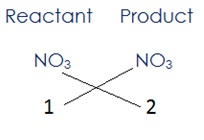Here Nitrogen and oxygen have been balanced, then Equation will be;

2HNO3 + Ca(OH)2 → Ca(NO3)2 + H2O

(ii)  Next calcium is already balanced, now we have to balance only hydrogen atoms.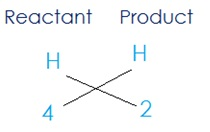Product need 2 as coefficient because (4 ÷ 2) = 2 , then equation will be;

2HNO3 + Ca (OH)2 → Ca(NO3)2 +2H2O

(iii)  On counting and listing the numbers of atom of different element that present in equation above.

Elements              Reactant                   Product

O                           8                                  8

N                           2                                  2

Ca                         1                                  1

H                            4                                  4

Hence we get a balanced equation

2HNO3 + Ca (OH)2 → Ca(NO3)2 +2H2O

I think now you have learnt how to balance a skeletal equation.

Important Study materials for classes 06, 07, 08,09,10, 11 and 12. Like CBSE Notes, Notes for Science, Notes for maths, Notes for Social Science, Notes for Accountancy, Notes for Economics, Notes for political Science, Noes for History, Notes For Bussiness Study, Physical Educations, Sample Papers, Test Papers, Mock Test Papers, Support Materials and Books.Mathematics Class - 11th

NCERT Maths book for CBSE Students.

books

## Study Materials List:

##### CBSE NOTES ⇒ Class 10th ⇒ Science
1. Chemical Reactions and Equations
2. Acids, Bases and Salts
3. Metals and Non-metals
4. Carbon and its Compounds
5. Periodic Classification of Elements
6. Life Processes
7. Control and Coordination
8. How do Organisms Reproduce
9. Heredity and Evolution
10. Light-Reflection and Refraction
11. Human Eye and Colourful World
12. Electricity
13. Magnetic Effects of Electric Current
14. Sources of Energy
15. Our Environment
16. Management of Natural Resources

New Books

See More ...